# Apple giving up on information technology

Apple has reinvented itself radically many times and done so when things were still going well. This is part of the companies success story. And the CEO Tim Cook apparently plans to continue with this strategy. Major reinventions where:

• Moving from the Apple II to Macintosh
• Dropping System 9 and replacing it by the totally new OS X
• Shifting the priority from computers to the i-pod
• Moving from i-pod to i-phone and i-pad

There were more, but these transformations could all be explained by early anticipation of an end or major shrink of the existing business. For example i-pods were nice music players, but then each phone contained a music player and every person had a phone in the pocket anyway, so the usefulness of an i-pod was tending to zero. Apple fans are very loyal, so they kept buying apple products, even when they were becoming obsolete, but that can only work for a short time. So they made a i-phone, which was theoretically a phone, but the phone-functionally did not really work. I assume, with more recent iphones they have fixed this.

But Tim Cook is moving on. What are the strengths of Apple? Design, marketing and sales. Especially including the fans in working for free for the company to enhance sales. This is an essential part of the success story. We are already used to the fact, that useful features are radically removed, like the DVD player in laptops, when DVDs were still important. Or the standard earphone plug from phones. Apple fans feel flattered by this, because they are so advanced by losing something first that later on actually becomes obsolete.

So the next step is coming now: Apple will move on to fashion. They will design clothes, shoes and jewelry. Then they can concentrate on their real strengths, reinvent themselves once more and remain in business for another long future. Computers, tablets and phones will be phased out.

# Skillsmatter working again

The original Skillsmatter company has gone into administration and will be closed down, once all claims have been settled.

Now investors have bought assets of this old company, apparently including the name and the brand, and are now operating und the same name and webdomain skillmatter.com. This is a new company with new management and a new location. Probably some employees of the old skillsmatter are working in the new company.

They plan to continue activities of the old skillsmatter, for example conferences.

This has some implications. If you have bought a ticket for a conference from skillsmatter and this conference did not take place, the new skillsmatter is not obliged to give a refund. This is the old skillsmatters or more precisely its administrators Resolvegroup (See FAQ #13).

The other option is to hope that they will reschedule the events, which they promise to do. They promise to honor tickets from old Skillsmatter, even though they do not have to.

Please, make a choice between the two options. If you get money back from Resolve, you cannot use the same ticket to go to a conference of the new Skillsmatter. It would be abusive and I assume that they will check.

Concerning the two conferences that I liked to visit from Skillsmatter, Scala Exchange and Clojure Exchange, alternatives have been created, Functional Scala and ReClojure. Functional Scala is there to stay, at least for the next couple of years. So it will compete with a potentially revived Scala Exchange. For 2020 I have opted for Functional Scala and already bought the ticket. It was also said that reClojure intends to continue as well, but I consider it possible that they will merge efforts with Clojure Exchange.

# Pagination of Database Query Results

We have a typical database base backed web application. It can be a rich client. It can be a NoSQL database. Whatever, but for the moment maybe a transactional SQL database and a web application will be used as the most common and best understood example.

We kind of construct a query, of which the result set is so large, that we do not want to transfer, render and display it all at once. Think of Google, where you might have millions of hits and only see the first 10 or 100 or so. Now you can navigate through the result set, usually by going to the next pages successively.

Nice web applications tell you how many records there are exactly. And then calculate how to split this up into a large number of pages and allowing you to go to an arbitrary page, or at least to some of the pages (last, next 10 pages) immediately.

There are several problems with this. First of all: the set you are basing this on changes with time. This could be fixed by creating some temporary snapshot in memory, in the database or in some kind of db-cache and keeping that around for some time. This is expensive and usually not worth the price, so we live with some misbehavior.

The second problem is the count. Count actually needs to do a full scan of the result set, there is no decent short cut. Now it would usually be good enough to estimate the size of the result set and that is exactly what google does. Sometimes the estimation is ridiculously bad, but we have gotten used to it. That is much better than using the real count, because it really speeds up the application by a great factor and improves user experience overall. Of course it is a bit more challenging to program an estimation and only worth it for big result sets. We could still jump to any of the next 10 pages by just increasing the size of the current query by 11 and showing the required segment. Since we sort the result by something, we could just get the first N results sorted backwards and then wrap that in a query that sorts them forward. So dropping the count can help for performance without too much loss in functionality.

Now the next problem is, that having navigated through a lot of pages, the usual way of doing a query and then constraining it with some mechanism like „rownum“, „row_number“, „limit/offset“ to the subset we want to display always requires obtaining the resultset from the beginning to our page. Usually that does not matter, because we navigate through the application manually and that means not too many pages from the beginning. But it can become an issue, if the application is heavily used.

Now we sort by something. Usually not the id, but some business logic relevant keys or a combination. They do not have to be unique, but we can always add the ID (or more generally the primary key) as the last sort key to make it unique. Then the next page can be found by just adding a condition based on our sort key. Assuming we want to show N records. The query might be
 SELECT * FROM TABX    WHERE cond1 AND cond2    ORDER BY A, B, C ASC    LIMIT n; 
Now the last record of the result has A=a1, B=b1, C=c1.
Then the next page can be obtained by
 SELECT * FROM TABX    WHERE cond1 AND cond2     AND (A>a1 OR A=a1 AND B>b1 OR A=a1 AND B=b1 AND C>c1)    ORDER BY A, B, C ASC    LIMIT n; 
To skip three pages and get the fourth of the following pages is done like this:
 SELECT * FROM TABX    WHERE cond1 AND cond2     AND (A>a1 OR A=a1 AND B>b1 OR A=a1 AND B=b1 AND C>c1)    ORDER BY A, B, C ASC   LIMIT n OFFSET 3*n; 
We do use (or simulate) OFFSET here, but in a more intelligent way, because we do not force it to work harder than necessary by rebasing our query on what we already know.

We get a bit more consistency, because new records being inserted in the beginning will not be seen, but they will at least not disturb the succession of our result set. Maybe that is worth more than the performance gain, because we get it with a reasonable effort. caches, snapshots or even keeping it in „session memory“ are not really serious approaches to this issue, they will just sooner or later blowup our application in production, at the worst possible moment.

# Perl Scripts for editing

Even though we do have IDEs with quite powerful refactoring mechanisms for many languages, it is still sometimes useful, to have another automated editing mechanism.

Why would that be the case?

Some examples:

In some cases there was an SQL script to create the database tables, which had been written first. From that classes and even CRUD operations in something like JDBC or DBI can be created. Even though most Java-projects that I have seen recently prefer using Hibernate (or more generally JPA2), there are some benefits in doing just plain old JDBC. This requires some discipline in writing the SQL in a uniform way and it was sometimes even necessary to have „magical comments“ that were ignored by the DB, but used by the script. This can save a lot of work and errors.

In some cases there were large numbers of HTML files. Now a similar kind of change had to be applied to each of them. Using a Script to parse the HTML file and to apply the changes can save a lot of work and provide consistency that is often badly needed. And if the whole thing happens in the context of an agile project, then the stakeholders might want to see the outcome and come up with further ideas. No big deal, just change the transformation scripts and check it out again. I recommend thinking in terms of larger transformation steps. Then I would retain the original files and apply the script for the step until the outcome is ok. Then this can be committed to git and the next step can be worked on. If the intermediate results are not useful for anybody else, just wait with the push or better work on a branch.

Sometimes refactorings have to be done that are not easily supported by the IDE. For example a whole bunch of classes need to be moved to different packages according to some new naming rule. Just find all the classes with their old package names, then move to the new directory structure and rename imports and package declarations to the new structure. This can easily be done with a Script. Always remember to be careful if there is Reflection involved, this will most likely break all refactorings, no matter if by IDE or by script.

Also it is often useful to use scripts to analyze code and to find occurrences of certain patterns.

These things can be done with scripts in Ruby, Python, Perl or Raku. All of these are valid options. Ruby and Raku are somewhat more sophisticated languages than the other two. And Perl and Raku have the most sophisticated Regex capabilities. I would assume that Raku is the best choice, if you start from scratch, it even supports grammars out of the box, which might be a way to address such issues. Perl has it as add on libraries. Of course it is also useful to work with what you know. But sometimes it is worth learning the right tool instead of just using the golden hammer to put in screws.

So in my case the tool for this is currently Perl. It does the job, is available more or less everywhere and I know it well enough. It might be worth moving to Raku in the future.

Some things that often work for simple scripts are the following:

Try to start with normalizing the input files, this might be the first transformation step. Remove trailing spaces, replace tabs with spaces, maybe normalize idention, replace line endings by LF only without CR. In HTML replace HTML-entities for UNICODE-characters by the appropriate Unicode characters, convert everything to UTF-8 etc.
If you have data like phone numbers and dates in the file that are meaningful for your further steps, bring them to a standard format. Of course always depending on your local circumstances, but this is usually the right way to go. This might be partially done with an external tool like xmllint.

For the next steps we need to consider the issue that we have multiple lines. There are regex-variants that do not stop at line boundaries. But if we read the content line wise, it can still be a bit more work. Sometimes it is easier to just replace line feeds by some marker string that does not otherwise occur in your files (check it before!) and then apply usual regex on this longer line.

What we often need is a regex that finds the shortest and not the longest match. If we write something like /a.*b/, this will look for a sequence that starts with an „a“ and ends with the last „b“ that can be found. Often we want to end with the first „b“. This can be achieved by /a.*?b/.

Another pattern that is often useful for such scripts is to work with a state machine. If a certain pattern is discovered, we react to it depending on the state and possibly change the state. So we can apply changes to something only if it occurs in a certain context.

# JSON instead of Java Serialization: The solution?

We start recognizing that Serialization is not such a good idea.

It is cool and can really work on a wide range of objects, even including complex and cyclic reference graphs. And it was essential for some older Java frameworks like EJB and RMI, which allowed remote access to Java objects and classes.

But it is no longer the future, Oracle will soon deprecate and later remove it. And it will happen this time, even though they really keep stuff around for a long time due to compatibility requirements.

Just to recap: it opens up security discussion, it opens up hidden behavior and makes it harder to reason about code, it creates tight coupling between remote components and it can result in bugs, that only occur at runtime and cannot be discovered at compile time. In short, it is not resilient.

So we need something else. Obvious candidates are XML, YAML and JSON. XML is of course an option and is powerful enough to do many things, but often a bit too clumbsy and too much boiler plate, so we try to move away from it. YAML and JSON kind of do the same thing, but it seems that JSON is winning the race and we all need to know JSON and many of us tend to skip YAML.

So why not use JSON. It is easy, it has good libraries and we can even find databases that work with JSON.

What JSON can express very well are scalars, lists and maps and combinations of these. This is quite exactly what we have in Perl, JavaScript or Clojure as basic building blocks. These languages support object oriented programming, but for simple stuff we go with these basic building blocks. And objects can be modelled as (hash-)maps, with the attribute names as keys. Actually JSON is valid JavaScript code.

We do have to change our thinking when moving from Java Serialization to JSON. JSON does not store any serializable object but just data. Maybe that is enough and that is what we actually want. It totally works in heterogeneous environments, where we are using different programming languages or different implementations.

There are good libraries. I have tried two, Jackson and GSON which both work well, recently mostly Jackson. It is important to think of Clojure, JavaScript, Perl or something like that without objects. So we loose type information, which can be considered good or bad, but if we can arrange ourselves with it, we avoid the tight coupling. JavaBeans are expressed exactly the same as a HashMap with the attribute names as keys. We can provide the top level class when deserializing, but at the child levels it will not be able to figure that out, if it relies on runtime information.

## Example Code

Here it has been tried out. Find full example code on github.

A class that contains all kinds of stuff. Not prepared for really putting in nulls, but it is just experimental code…

 package net.itsky.jackson;

 import java.util.List; import java.util.Map; import java.util.Set; public class TestObject {     private Long l;     private String s;     private Boolean b;     private Set set;     private List list;     private Map map;     public TestObject(Long l, String s, Boolean b, Set set, List list, Map map) {         this.l = l;         this.s = s;         this.b = b;         this.set = set;         this.list = list;         this.map = map;     }     public TestObject() {         // only for framework purposes     }     public Long getL() {         return l;     }     public String getS() {         return s;     }     public Boolean getB() {         return b;     }     public Set getSet() {         return set;     }     public List getList() {         return list;     }     public Map getMap() {         return map;     } 

    @Override     public String toString() {         return getClass().getSimpleName() + "(" +                "l=" + l + " (" + l.getClass() + ") "                 + " s=\"" + s + "\" (" + s.getClass() + ") "                 + " b=" + b + " (" + b.getClass() + ") "                 + " set=" + set  + " (" + set.getClass() + ") "                 + " list=" + list + " (" + list.getClass() + ") "                 + " map=" + map + " (" + map.getClass() + "))";     } } 
And this is used for running everything. To play around more, it should probably be moved to tests..
 package net.itsky.jackson;

 import com.fasterxml.jackson.databind.ObjectMapper; import com.fasterxml.jackson.databind.ObjectWriter; import com.google.common.collect.ImmutableList; import com.google.common.collect.ImmutableMap; import com.google.common.collect.ImmutableSet; import java.io.StringReader; import java.util.List; import java.util.Map; import java.util.Set; public class App { 

    public static void main(String[] args) {         try {             Set s1 = ImmutableSet.of(1, 2, 3);             Set s2 = ImmutableSet.of(1, 2, 3);             Map m1 = ImmutableMap.of("A", "abc", "B", 3L, "C", s1);             List l1 = ImmutableList.of("i", "e", "a", "o", "u");             TestObject t1 = new TestObject(30303L, "uv", true, s2, l1, m1);             Map m2 = ImmutableMap.of("r", "r101", "s", 202, "t", t1);             List l2 = ImmutableList.of("ä", "ö", "ü", "å", "ø");             Set s3 = ImmutableSet.of("x", "y", "z");             TestObject t2 = new TestObject(40404L, "ijk", false, s3, l2, m2);             ObjectMapper mapper = new ObjectMapper();             ObjectWriter writer = mapper.writerWithDefaultPrettyPrinter();             System.out.println("t2=" + t2);             String json = writer.writeValueAsString(t2);             System.out.println("json=" + json);             StringReader stringReader = new StringReader(json);             TestObject t3 = mapper.readValue(stringReader, TestObject.class);             System.out.println("t3=" + t3);         } catch (Exception ex) {             RuntimeException rex;             if (ex instanceof RuntimeException) {                 rex = (RuntimeException) ex;             } else {                 rex = new RuntimeException(ex);             }             throw rex;         }     } } 
And here is the output:
 t2=TestObject(l=40404 (class java.lang.Long)  s="ijk" (class java.lang.String)   b=false (class java.lang.Boolean)   set=[x, y, z] (class com.google.common.collect.RegularImmutableSet)   list=[ä, ö, ü, å, ø] (class com.google.common.collect.RegularImmutableList)   map={r=r101, s=202,  t=TestObject(l=30303 (class java.lang.Long)   s="uv" (class java.lang.String)  b=true (class java.lang.Boolean)   set=[1, 2, 3] (class com.google.common.collect.RegularImmutableSet)   list=[i, e, a, o, u] (class com.google.common.collect.RegularImmutableList)   map={A=abc, B=3, C=[1, 2, 3]} (class com.google.common.collect.RegularImmutableMap))}  (class com.google.common.collect.RegularImmutableMap)) json={   "l" : 40404,   "s" : "ijk",   "b" : false,   "set" : [ "x", "y", "z" ],   "list" : [ "ä", "ö", "ü", "å", "ø" ],   "map" : {     "r" : "r101",     "s" : 202,     "t" : {       "l" : 30303,       "s" : "uv",       "b" : true,       "set" : [ 1, 2, 3 ],       "list" : [ "i", "e", "a", "o", "u" ],       "map" : {         "A" : "abc",         "B" : 3,         "C" : [ 1, 2, 3 ]       }     }   } } t3=TestObject(l=40404 (class java.lang.Long)  s="ijk" (class java.lang.String)   b=false (class java.lang.Boolean)   set=[x, y, z] (class java.util.HashSet)   list=[ä, ö, ü, å, ø] (class java.util.ArrayList)   map={r=r101, s=202,  t={l=30303, s=uv, b=true,  set=[1, 2, 3],  list=[i, e, a, o, u],  map={A=abc, B=3, C=[1, 2, 3]}}}   (class java.util.LinkedHashMap))

 

Process finished with exit code 0 

So the immediate object and its immediate attributes were deserialized properly to what we provided. But everything inside went to maps, lists and scalars.

The intermediate JSON does not carry the type information at all, so this is the best that can be done.
Often it is useful what we want. If not, we need to find something else or see if we can tweak JSON to carry type information.

It will be interesting to explore other serialization protocols…

# Project-local Libraries

Many projects have these „project-local“ or „company-local“ libraries, that can be used optionally or are even strongly imposed on developers. They may be called something like

• core
• toolkit
• toolbox
• base
• platform
• utils
• lib
• common
• framework
• baselib
• sdk
• tools

or even with a meaningful good name.

Generally there is nothing wrong with such libraries, if they are used correctly.

Some things should be observed, though: Such libraries are there to be useful. If that is not the case, it is better not to use them and to deprecate and discard them. Quite a lot of projects suffer from the fact that inferior local libraries are imposed on them and have to be used. The strive for „consistency“ is not bad either, but it should be kept to a level at which it is useful and not become a primary goal by itself.

So when need for a functionality arises, an existing library might cover this well enough. If it is not too hard to integrate, there is little need to write a „local library functionality“ for this. If no good library can be found or reasonably be integrated, it is a good idea to write what is needed oneself. It should be observed, that such a library should have slightly higher quality standards and very good automated testing, because it is more universal and the actual and future usage cannot be anticipated as easily as with „business code“. There are places, where it is good to impose a local library, to make sure that certain fields are validated in the same way across the software or even across the organization, for example. For data like phone numbers, dates, email addresses etc. that are commonly used in our world, it is probably possible to find good libraries that do this much better than any local library with reasonable team effort could do.

Now the world moves and we might discover better replacements for parts of the local library. It is a good idea to move on to these better replacements.

Things that can go into a local library are small pieces of business logic that need to be used everywhere. Think of an insurance company. Customers have customer numbers. They have to be entered into web application forms and they have to be checked for correctness, they have to be formatted etc. This could go into a library and we could be sure that everyone in the larger project uses the same rules for what is a valid customer number and how to format and parse them. It is a bit too hard to implement it again and again, because tiny errors sneak in, for example into the code to check for validity with some check sum, but it is too easy to put it into a service that can be called to check formal validity. Such a service will be there to check if some formally correct customer number is actually a real customer’s number, maybe with constraints on who can see this for which customer. And of course to generate new customer numbers, when needed.

It is always good to ask twice if certain functionality should rather sit in a service that can be called or in a library, of course depending also on the local architecture preferences. In cases where the functionality is useful for others in the organization, but where the exact same behavior is less important, it can also be an option to just copy the code to different teams. But this should be used with care, because it means that improvements do not easily flow back to benefit other users of the functionality.

Generally it is an observation that organizations rarely have good „local libraries“. The good libraries are found on the internet. Or only exist within the team. And the bad company libraries are often forced on those who cannot find ways to opt out. Good team libraries that are not known outside of the team can sometimes be a loss for the organization, because things are done twice or even worse are done differently where it would be good to have the same behavior.

# Perl and Scala: what can they learn from each other?

Ironically Scala at first drew my interest, because I discovered that about ten years ago there was no really good understanding of how to do a good multithreading concept for Perl 6. I thought exploring how they do it in Scala, where it was already known to be good at that time, would give a more general understanding to this issue. At this time Perl 6 (now named „Raku“) was intended to rather go without multithreading capabilities than doing them badly. In the end I got dragged into Scala and found that by itself more interesting than the original issue. And Perl 6 community eventually found good answers for providing multithreaded capabilities anyway.

So why there are technical concepts in both of these languages, that are interesting and possibly could in some way be applied to the other one, there is an interesting parallel.

Both Scala and Perl have been „cool languages“ that were really strong in an area or even in a broader range of application areas. Both of them found a competitor, that was kind of an „inferior clone“ of them. PHP in its early versions was very similar to Perl, but „simplified“ and kind of a subset of what Perl provided. At that time Perl had a real boom, because the first Web applications came up and the only reasonable way to go was CGI and of course it was done with Perl. There were some early alternatives like Cold Fusion and ASP, but they never really become main stream, at least not outside of their respective communities. Now PHP eventually took over most of Perl’s CGI and has become a major building block of our current WWW. Wikipedia and this Blog run on PHP. Perl eventually also lost its leading position as system administration scripting language to Ruby and even more to Python and some others, but it is still there and has strong string parsing capabilities and a very useful ecosystem of libraries called CPAN.

Now Scala has found Kotlin to be a similar competitor. Besides being somewhat simpler Kotlin also shines with good tooling support. It comes from the same organization as IntelliJ IDEA, which is the usual IDE for most JVM-languages for people who rely neither on Emacs nor vi. So Kotlin support in IntelliJ is always going to be a high priority. And Kotlin is officially supported by Google as programming language for Android-apps. It seems to work well, allows for more modern development than the supported Java versions and has conceptionally a lot of similarity with Swift, which is the most modern programming language supported by Apple for IOS-Apps. There have been heroic and admirable approaches to allow for Android App development using other JVM-languages, especially Scala. But they all suffer from the same set of problems. In order to avoid installing too much language specific code in the app, dynamic language features that would require a compile capability, as commonly used for Groovy or Clojure have to be avoided. And the excessive use of the languages libraries has to be avoided, because they are not on the phone already, but a copy of them has to be shipped with the app, for each App. So the storage usage is much more than for Kotlin and Java Apps. And then we see an attempt, to reduce the size of the libraries, by only including what is needed. That is necessary, but it looks too fragile to really trust it. So, for Mobile Apps, it is Kotlin. Period. And then Kotlin is already there, so why not use it on the server as well. Yes, I do believe Scala is better, but that is not what everyone thinks and it needs to be much better to justify the additional language, where App-development for Android is already happening.

Now both Perl and Scala had some problems. To some extent, they are even sharing the exact same problem. It was the possibility to write really „cool“ code that was very smart, very short and could not be read by anybody else without very much time and very much knowledge. This can be done in any language, but Perl is the number one for this and I would put Scala as number two and C++ and C as number three and four, from the languages, that I know. It is a good idea to use some coding standards that allow for clean Scala or Perl code. But please remain reasonable and do not let bureaucrats come in charge of the coding standards to create a monster that drains all creativity. Allow using powerful features, but use them in a decent and readable way.

Now in both cases, there was an effort, to write a new version of the language, that was meant to be slightly incompatible and cleaned up some of the weaknesses and brought some improvements. In case of Perl this was Perl 6. It was developed for around 20 years and came out a few years ago. Eventually it turned out too different, so it was renamed to Raku. For Scala, a new language called „Dotty“ was developed. It was decided to make this the next major version, Scala 3. Even though it is much closer to Scala 2 than Raku to Perl 5, it is still incompatible and requires an effort to rewrite code. It is already seen that large Perl 5 projects are hardly moving to Raku, so Perl 5 is there to stay and Raku is just a second language within the same community. This will probably not happen like that with Scala, and the core language team will probably at some point of time concentrate on Scala 3. But large organizations that heavily invested into Scala cannot easily migrate, simply because it needs a lot of time and money. So we will probably also see some long term coexistence of Scala 2 and Scala 3. Maybe Scala 2 will be forked by major adopters. Or it will be supported for money from Lightbend.

# Phone Numbers and E-Mail Addresses

Most data that we deal with are strings or numbers or booleans and combinations of these into classes and collections. Dates can be expressed as string or number, but have enough specific logic to be seen as a fourth group of data. All these have interesting aspects, some of which have been discussed in this blog already.

Now phone numbers are by an naïve approach numbers or strings, but very soon we see that they have their own specific aspects. The same applies for email addresses which can be represented as strings.

Often projects go by their own „simplified“ specification of what an email address or a phone number is, how to parse, compare and render them. In the end of the day the simplification is harder to tame than the real solution, because it needs to be maintained and specified by the project team rather than being based on a proven library. And once in a while „edge cases“ occur, that cannot be ignored and that make the „home grown“ library even more complex.

Behind phone numbers and email addresses there are well defined and established standards and they are hard to understand thoroughly within the constrained time budget of a typical „business project“, because the time should be allocated to enhancing the business logic and not to reinventing the basics. Unless there is a real need to do so, of course.

Just to give an idea: When phone numbers are parsed or provided by user input, they can start with a „+“ sign or use some country specific logic to express, to which country they belong. And then the „+1“, for example, does not stand for the United States alone, but also for Canada and some smaller countries that are in some way associated with the United States or Canada. Further analysis of the number is required to know about that. The prefix for international number is often „00“, but in the United States it is „011“ and there were and are some other variants, that are still frequently used. Some people like to write something like „+49(0)431 77 88 99 11 1“ instead of „+49 431 77 88 99 11 1“. We can constrain the input to the variants we happen to think of and force the supplier of data to comply, but why bother? Why not accept legitimate formats, as long as they are correct and unambiguous?

Now for E-Mail-addresses there is the famous one page regular expression to recognize correct email addresses which is even by itself not totally complete. Find it at the bottom of the article…

Of course it includes some rarely used variants of email addresses that were once used and have not been completely abolished officially, but it is hard to draw and exact border for this.

So the general recommendation is to find a good library for working with email addresses and phone numbers. Maybe the library can even to some extent eliminate input strings that are formally complying the format, but know to be incorrect by knowing about numbering schemes world wide or about email domains or even by performing lookups.

Another strong recommendation is to store data like email addresses and phone numbers in a technical format, that is in the example of phone numbers always starting with a „+“ followed by digits only. For input any positioning of spaces is accepted, for output the library knows how to format it correctly. This allows selecting by the numbers without dealing with complex formatting, by just using the technical format in the query as well.

For Java (and thus for many JVM-languages), C++ and JavaScript there is an excellent library from Google for dealing with phone numbers. For E-Mails something like apache commons email validator is a way to go.

Keep in mind that for E-Mail addresses and phone numbers, the ultimate way of verification is to send them a link or a code that they need to enter. In the end of the day it is insufficient to rely only on formal verification without this final step.

But still issues remain for transforming data into a canonical technical format for storing them, formatting data for display etc. And there is a huge added value, if we can reliably recognize formally false entries early, when the user can still easily react to it, rather than waiting for an email/SMS/phone call being processed, which may fail when the user is no longer on our „registration site“. And we can process data which has already been verified by a third party, but still we want to parse it to recognize obvious errors.

The concrete libraries may be outdated by the time you are reading this, or they may not be applicable for the language environment that you are using, but please make an effort to find something similar.

So, please use good libraries, that are like to be found for the environment that you are using and write yourself what creates value for your project or organization. Unless your goal is really to write a better library. Better invest the time into areas where there are still no good libraries around.

And as always, you may understand email addresses and phone numbers as an example for a more general idea.

## E-Mail Regex

(?:(?:\r\n)?[ \t])*(?:(?:(?:[^()<>@,;:\\". \000-\031]+(?:(?:(?:\r\n)?[ \t] )+|\Z|(?=[$"()<>@,;:\\".\[$]))|"(?:[^\"\r\\]|\\.|(?:(?:\r\n)?[ \t]))*"(?:(?: \r\n)?[ \t])*)(?:\.(?:(?:\r\n)?[ \t])*(?:[^()<>@,;:\\". \000-\031]+(?:(?:( ?:\r\n)?[ \t])+|\Z|(?=[$"()<>@,;:\\".\[$]))|"(?:[^\"\r\\]|\\.|(?:(?:\r\n)?[ \t]))*"(?:(?:\r\n)?[ \t])*))*@(?:(?:\r\n)?[ \t])*(?:[^()<>@,;:\\". \000-\0 31]+(?:(?:(?:\r\n)?[ \t])+|\Z|(?=[$"()<>@,;:\\".\[$]))|$([^\[$\r\\]|\\.)*\ ](?:(?:\r\n)?[ \t])*)(?:\.(?:(?:\r\n)?[ \t])*(?:[^()<>@,;:\\". \000-\031]+ (?:(?:(?:\r\n)?[ \t])+|\Z|(?=[$"()<>@,;:\\".\[$]))|$([^\[$\r\\]|\\.)*\](?: (?:\r\n)?[ \t])*))*|(?:[^()<>@,;:\\". \000-\031]+(?:(?:(?:\r\n)?[ \t])+|\Z |(?=[$"()<>@,;:\\".\[$]))|"(?:[^\"\r\\]|\\.|(?:(?:\r\n)?[ \t]))*"(?:(?:\r\n) ?[ \t])*)*\<(?:(?:\r\n)?[ \t])*(?:@(?:[^()<>@,;:\\". \000-\031]+(?:(?:(?:\ r\n)?[ \t])+|\Z|(?=[$"()<>@,;:\\".\[$]))|$([^\[$\r\\]|\\.)*\](?:(?:\r\n)?[ \t])*)(?:\.(?:(?:\r\n)?[ \t])*(?:[^()<>@,;:\\". \000-\031]+(?:(?:(?:\r\n) ?[ \t])+|\Z|(?=[$"()<>@,;:\\".\[$]))|$([^\[$\r\\]|\\.)*\](?:(?:\r\n)?[ \t] )*))*(?:,@(?:(?:\r\n)?[ \t])*(?:[^()<>@,;:\\". \000-\031]+(?:(?:(?:\r\n)?[ \t])+|\Z|(?=[$"()<>@,;:\\".\[$]))|$([^\[$\r\\]|\\.)*\](?:(?:\r\n)?[ \t])* )(?:\.(?:(?:\r\n)?[ \t])*(?:[^()<>@,;:\\". \000-\031]+(?:(?:(?:\r\n)?[ \t] )+|\Z|(?=[$"()<>@,;:\\".\[$]))|$([^\[$\r\\]|\\.)*\](?:(?:\r\n)?[ \t])*))*) *:(?:(?:\r\n)?[ \t])*)?(?:[^()<>@,;:\\". \000-\031]+(?:(?:(?:\r\n)?[ \t])+ |\Z|(?=[$"()<>@,;:\\".\[$]))|"(?:[^\"\r\\]|\\.|(?:(?:\r\n)?[ \t]))*"(?:(?:\r \n)?[ \t])*)(?:\.(?:(?:\r\n)?[ \t])*(?:[^()<>@,;:\\". \000-\031]+(?:(?:(?: \r\n)?[ \t])+|\Z|(?=[$"()<>@,;:\\".\[$]))|"(?:[^\"\r\\]|\\.|(?:(?:\r\n)?[ \t ]))*"(?:(?:\r\n)?[ \t])*))*@(?:(?:\r\n)?[ \t])*(?:[^()<>@,;:\\". \000-\031 ]+(?:(?:(?:\r\n)?[ \t])+|\Z|(?=[$"()<>@,;:\\".\[$]))|$([^\[$\r\\]|\\.)*\]( ?:(?:\r\n)?[ \t])*)(?:\.(?:(?:\r\n)?[ \t])*(?:[^()<>@,;:\\". \000-\031]+(? :(?:(?:\r\n)?[ \t])+|\Z|(?=[$"()<>@,;:\\".\[$]))|$([^\[$\r\\]|\\.)*\](?:(? :\r\n)?[ \t])*))*\>(?:(?:\r\n)?[ \t])*)|(?:[^()<>@,;:\\". \000-\031]+(?:(? :(?:\r\n)?[ \t])+|\Z|(?=[$"()<>@,;:\\".\[$]))|"(?:[^\"\r\\]|\\.|(?:(?:\r\n)? [ \t]))*"(?:(?:\r\n)?[ \t])*)*:(?:(?:\r\n)?[ \t])*(?:(?:(?:[^()<>@,;:\\". \000-\031]+(?:(?:(?:\r\n)?[ \t])+|\Z|(?=[$"()<>@,;:\\".\[$]))|"(?:[^\"\r\\]| \\.|(?:(?:\r\n)?[ \t]))*"(?:(?:\r\n)?[ \t])*)(?:\.(?:(?:\r\n)?[ \t])*(?:[^()<> @,;:\\". \000-\031]+(?:(?:(?:\r\n)?[ \t])+|\Z|(?=[$"()<>@,;:\\".\[$]))|" (?:[^\"\r\\]|\\.|(?:(?:\r\n)?[ \t]))*"(?:(?:\r\n)?[ \t])*))*@(?:(?:\r\n)?[ \t] )*(?:[^()<>@,;:\\". \000-\031]+(?:(?:(?:\r\n)?[ \t])+|\Z|(?=[$"()<>@,;:\\ ".\[$]))|$([^\[$\r\\]|\\.)*\](?:(?:\r\n)?[ \t])*)(?:\.(?:(?:\r\n)?[ \t])*(? :[^()<>@,;:\\". \000-\031]+(?:(?:(?:\r\n)?[ \t])+|\Z|(?=[$"()<>@,;:\\".\[$]))|$([^\[$\r\\]|\\.)*\](?:(?:\r\n)?[ \t])*))*|(?:[^()<>@,;:\\". \000- \031]+(?:(?:(?:\r\n)?[ \t])+|\Z|(?=[$"()<>@,;:\\".\[$]))|"(?:[^\"\r\\]|\\.|( ?:(?:\r\n)?[ \t]))*"(?:(?:\r\n)?[ \t])*)*\<(?:(?:\r\n)?[ \t])*(?:@(?:[^()<>@,; :\\". \000-\031]+(?:(?:(?:\r\n)?[ \t])+|\Z|(?=[$"()<>@,;:\\".\[$]))|$([ ^\[$\r\\]|\\.)*\](?:(?:\r\n)?[ \t])*)(?:\.(?:(?:\r\n)?[ \t])*(?:[^()<>@,;:\\" . \000-\031]+(?:(?:(?:\r\n)?[ \t])+|\Z|(?=[$"()<>@,;:\\".\[$]))|$([^\[\ ]\r\$|\\.)*\](?:(?:\r\n)?[ \t])*))*(?:,@(?:(?:\r\n)?[ \t])*(?:[^()<>@,;:\\".\ [\] \000-\031]+(?:(?:(?:\r\n)?[ \t])+|\Z|(?=[$"()<>@,;:\\".\[$]))|$([^\[$\ r\\]|\\.)*\](?:(?:\r\n)?[ \t])*)(?:\.(?:(?:\r\n)?[ \t])*(?:[^()<>@,;:\\". \000-\031]+(?:(?:(?:\r\n)?[ \t])+|\Z|(?=[$"()<>@,;:\\".\[$]))|$([^\[$\r\\] |\\.)*\](?:(?:\r\n)?[ \t])*))*)*:(?:(?:\r\n)?[ \t])*)?(?:[^()<>@,;:\\". \0 00-\031]+(?:(?:(?:\r\n)?[ \t])+|\Z|(?=[$"()<>@,;:\\".\[$]))|"(?:[^\"\r\\]|\\ .|(?:(?:\r\n)?[ \t]))*"(?:(?:\r\n)?[ \t])*)(?:\.(?:(?:\r\n)?[ \t])*(?:[^()<>@, ;:\\". \000-\031]+(?:(?:(?:\r\n)?[ \t])+|\Z|(?=[$"()<>@,;:\\".\[$]))|"(? :[^\"\r\\]|\\.|(?:(?:\r\n)?[ \t]))*"(?:(?:\r\n)?[ \t])*))*@(?:(?:\r\n)?[ \t])* (?:[^()<>@,;:\\". \000-\031]+(?:(?:(?:\r\n)?[ \t])+|\Z|(?=[$"()<>@,;:\\". \[$]))|$([^\[$\r\\]|\\.)*\](?:(?:\r\n)?[ \t])*)(?:\.(?:(?:\r\n)?[ \t])*(?:[ ^()<>@,;:\\". \000-\031]+(?:(?:(?:\r\n)?[ \t])+|\Z|(?=[$"()<>@,;:\\".\[$ ]))|$([^\[$\r\\]|\\.)*\](?:(?:\r\n)?[ \t])*))*\>(?:(?:\r\n)?[ \t])*)(?:,\s*( ?:(?:[^()<>@,;:\\". \000-\031]+(?:(?:(?:\r\n)?[ \t])+|\Z|(?=[$"()<>@,;:\\ ".\[$]))|"(?:[^\"\r\\]|\\.|(?:(?:\r\n)?[ \t]))*"(?:(?:\r\n)?[ \t])*)(?:\.(?:( ?:\r\n)?[ \t])*(?:[^()<>@,;:\\". \000-\031]+(?:(?:(?:\r\n)?[ \t])+|\Z|(?=[ $"()<>@,;:\\".\[$]))|"(?:[^\"\r\\]|\\.|(?:(?:\r\n)?[ \t]))*"(?:(?:\r\n)?[ \t ])*))*@(?:(?:\r\n)?[ \t])*(?:[^()<>@,;:\\". \000-\031]+(?:(?:(?:\r\n)?[ \t ])+|\Z|(?=[$"()<>@,;:\\".\[$]))|$([^\[$\r\\]|\\.)*\](?:(?:\r\n)?[ \t])*)(? :\.(?:(?:\r\n)?[ \t])*(?:[^()<>@,;:\\". \000-\031]+(?:(?:(?:\r\n)?[ \t])+| \Z|(?=[$"()<>@,;:\\".\[$]))|$([^\[$\r\\]|\\.)*\](?:(?:\r\n)?[ \t])*))*|(?: [^()<>@,;:\\". \000-\031]+(?:(?:(?:\r\n)?[ \t])+|\Z|(?=[$"()<>@,;:\\".\[\ ]]))|"(?:[^\"\r\$|\\.|(?:(?:\r\n)?[ \t]))*"(?:(?:\r\n)?[ \t])*)*\<(?:(?:\r\n) ?[ \t])*(?:@(?:[^()<>@,;:\\". \000-\031]+(?:(?:(?:\r\n)?[ \t])+|\Z|(?=[$" ()<>@,;:\\".\[$]))|$([^\[$\r\\]|\\.)*\](?:(?:\r\n)?[ \t])*)(?:\.(?:(?:\r\n) ?[ \t])*(?:[^()<>@,;:\\". \000-\031]+(?:(?:(?:\r\n)?[ \t])+|\Z|(?=[$"()<> @,;:\\".\[$]))|$([^\[$\r\\]|\\.)*\](?:(?:\r\n)?[ \t])*))*(?:,@(?:(?:\r\n)?[ \t])*(?:[^()<>@,;:\\". \000-\031]+(?:(?:(?:\r\n)?[ \t])+|\Z|(?=[$"()<>@, ;:\\".\[$]))|$([^\[$\r\\]|\\.)*\](?:(?:\r\n)?[ \t])*)(?:\.(?:(?:\r\n)?[ \t] )*(?:[^()<>@,;:\\". \000-\031]+(?:(?:(?:\r\n)?[ \t])+|\Z|(?=[$"()<>@,;:\\ ".\[$]))|$([^\[$\r\\]|\\.)*\](?:(?:\r\n)?[ \t])*))*)*:(?:(?:\r\n)?[ \t])*)? (?:[^()<>@,;:\\". \000-\031]+(?:(?:(?:\r\n)?[ \t])+|\Z|(?=[$"()<>@,;:\\". \[$]))|"(?:[^\"\r\\]|\\.|(?:(?:\r\n)?[ \t]))*"(?:(?:\r\n)?[ \t])*)(?:\.(?:(?: \r\n)?[ \t])*(?:[^()<>@,;:\\". \000-\031]+(?:(?:(?:\r\n)?[ \t])+|\Z|(?=[$"()<>@,;:\\".\[$]))|"(?:[^\"\r\\]|\\.|(?:(?:\r\n)?[ \t]))*"(?:(?:\r\n)?[ \t]) *))*@(?:(?:\r\n)?[ \t])*(?:[^()<>@,;:\\". \000-\031]+(?:(?:(?:\r\n)?[ \t]) +|\Z|(?=[$"()<>@,;:\\".\[$]))|$([^\[$\r\\]|\\.)*\](?:(?:\r\n)?[ \t])*)(?:\ .(?:(?:\r\n)?[ \t])*(?:[^()<>@,;:\\". \000-\031]+(?:(?:(?:\r\n)?[ \t])+|\Z |(?=[$"()<>@,;:\\".\[$]))|$([^\[$\r\\]|\\.)*\](?:(?:\r\n)?[ \t])*))*\>(?:( ?:\r\n)?[ \t])*))*)?;\s*)

# Ranges of Dates and Times

In Software we often deal with ranges of dates and times.

Let us look at it from the perspective of an end user.

When we say something like „from 2020-03-07 to 2019-03-10“ we mean the set of all timestampssuch that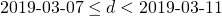or more accurately: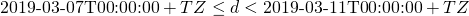Important is, that we mean to include the whole 24 hour day of 2019-03-10. Btw. please try to get used to the ISO-date even when writing normal human readable texts, it just makes sense…

Now when we are not talking about dates, but about times or instants of time, the interpretation is different.
When we say sonmething like „from 07:00 to 10:00“ or „from 2020-03-10T07:00:00+TZ to 2020-04-11T09:00:00+TZ“, we actually mean the set of all timestampssuch thator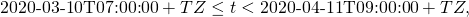respectively. It is important that we have to add one in case of date only (accuracy to one day) and we do not in case of finer grained date/time information. The question if the upper bound is included or not is not so important in our everyday life, but it proves that commonly the most useful way is not to include the upper bound. If you prefer to have all options, it is a better idea to employ an interval library, i.e. to find one or to write one. But for most cases it is enough to exclude the upper limit. This guarantees disjoint adjacent intervals which is usually what we want. I have seen people write code that adds 23:59:59.999 to a date and compares with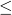instead of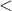, but this is an ugly hack that needs a lot of boiler plate code and a lot of time to understand. Use the exclusive upper limit, because we have it.

Now the requirement is to add one day to the upper limit to get from the human readable form of date-only ranges to something computers can work with. It is a good thing to agree on where this transformation is made. And to do it in such a way that it even behaves correctly on those dates where daylight saving starts or ends, because adding one day might actually mean „23 hours“ or „25 hours“. If we need to be really very accurate, sometimes switch seconds need to be added.

Just another issue has come up here. Local time is much harder than UTC. We need to work with local time on all kinds of user interfaces for humans, with very few exceptions like for pilots, who actually work with UTC. But local date and time is ambiguous for one hour every year and at least a bit special to handle for these two days where daylight saving starts and ends. Convert dates to UTC and work with that internally. And convert them to local date on all kinds of user interfaces, where it makes sense, including documents that are printed or provided as PDFs, for example. When we work with dates without time, we need to add one day to the upper limit and then round it to the nearest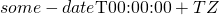for our timezone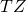or know when to add 23, 24 or 25 hours, respectively, which we do not want to know, but we need to use modern time libraries like the java.time.XXX stuff in Java, for example.

Working with date and time is hard. It is important to avoid making it harder than it needs to be. Here some recommendations:

• Try to use UTC for the internal use of the software as much as possible
• Use local date or time or date and time in all kinds of user interfaces (with few exceptions)
• add one day to the upper limit and round it to the nearest midnight of local time exactly once in the stack
• exclude the upper limit in date ranges
• Use ISO-date formats even in the user interfaces, if possible

# Functional Scala London 2019

In December 2019 I attended the conference Functional Scala in London which was initiated and managed by John de Goes. See Skillsmatter about what happened to Scala Exchange. Of course a large part of the conference was related to ZIO, which seems to be a part of the eco system surrounding Scala with a lot of dynamic.

It was a single track conference with a lot of talks, so I have attended all of them:
Day 1 (2019-12-12)

• KEYNOTE: XS — A Collections CLI [Paul Phillips] (Video)
• Introduction to Interruption [Jakub Kozlowski] (Video)
• Making Algorthms work with Functional Scala [Karl Brodowsky] (Video)
• Solving the Scala Notebook Experience [Jeremy Smith & Jonathan Indig] (Video)
• Mixing Scala & Kotlin [Alexey Soshin] (Video)
• Prototyping the Future with Functional Scala [Mike Kotsur] (Video)
• Test Effects: First Class [Adam Fraser] (Video)
• Let’s Gossip! [Dejan Mijic & Przemyslaw Wierzbicki] (Video)
• Ray Tracing with ZIO [Pierangelo Cecchetto] (Video)
• Invertible Programs [Sergei Shabanau] (Video)
• Hyper-pragmatic Pure FP Testing with DIStage-Testkit [Pavel Shirshov & Kai] (Video)
• KEYNOTE: Unleash Your Fury [Jon Pretty] (Video)

Day 2 (2019-12-14)

• Modern Data-Driven Applications with ZIO Streams [Itamar Ravid] (Video)
• Functional Architecture [Piotr Golebiewski] (Video)
• ZIO Chunk: A Fast, Pure Alternative to Arrays [Aleksandra A. Holubitska]
• Caliban: Designing a Functional GraphQL Library [Pierre Ricadat] (Video)
• Macros and Environmental Effects [Maxim Schuwalow] (Video)
• Streaming Analytics with Scala and Spark [Bas Geerdink] (Video)
• ZIO Actors [Mateusz Sokol] (Video)
• Adventures in Type-safe Error Handling [Jacob Wang]
• Composition using Arrows and Monoidal Categories [Oleg Nizhnik]
• Practical Logic(al) Programming with Dotty [Lander Lopez]
• Next-Level Type Safety: An Intro to Generalized Algebraic Data Types [Matthias Berndt]
• KEYNOTE: The Many Faces of Modularity [Eric Torreborre]

See Agenda

Maybe I will write more about some topics.

Talks will be on youtube in the near future.